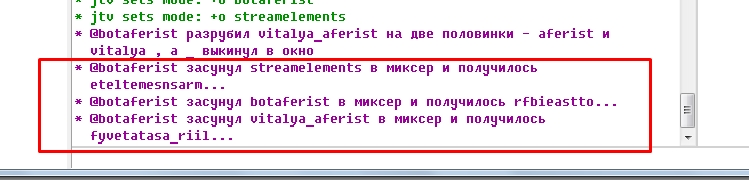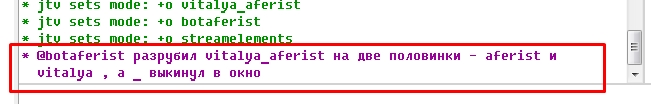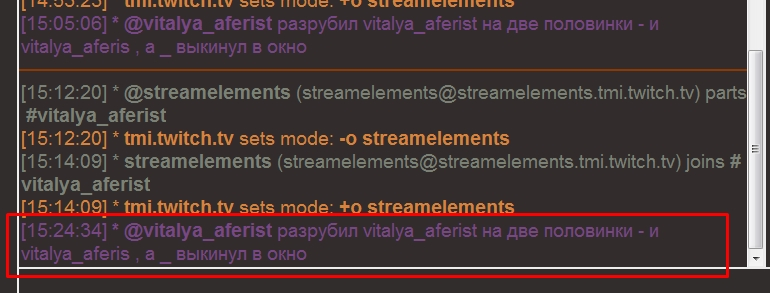## [Help] problems with mirc script

I know that there are problems with \$calc but here I have two scripts, and in one everything is good, and in another problem, I do not know why
in the first script everything is fine (do not look at Russian words), he mixes the letters in the wordmircadiirc

``````mixer {
var %t = \$1, %was
while (%t != \$null) {
var %x = \$rand(1,\$len(%t))
var %was = %was \$+ \$mid(%t,%x,1)
%t = \$left(%t,\$calc(%x - 1)) \$+ \$right(%t,\$calc(\$len(%t) - %x))
}
return %was
}

``````

and the second in mirc normally works, but in adiirc problemsmirc

here the script cuts the nickname ))
and if in the nickname there is _ then the script divides its right side puts in the beginning, and the left one to the right and _ throws it out the window))adiirc

but here the right part is not taken at all, and the left one takes all up to the penultimate character, but at the same time _ is discarded normally

``````popolam {
; если длина ника равна 1 букве
if (\$len(\$1) == 1) { return me расчленил \$\$1 на много половинок }
; если длина больше 2 и точка содержится при делении длины на 2, т.е. мы проверяем четное слово или нет
if (\$len(\$1) > 2) && (\$chr(46) isin \$calc(\$len(\$\$1) / 2)) { return me разрубил \$\$1 на две половинки - \$right(\$\$1,\$calc(\$len(\$\$1) / 2)) и \$left(\$\$1,\$calc(\$len(\$\$1) / 2)) , a \$mid(\$1,\$int(\$calc((\$len(\$1) /2)+1)),1) выкинул в окно }
; если число четное
else { return me разрубил \$\$1 на две половинки - \$right(\$\$1,\$calc(\$len(\$\$1) / 2)) и \$left(\$\$1,\$calc(\$len(\$\$1) / 2)) }
}

``````

what can be the problem and how to fix
Thanks!

### Replies (2)

#### RE: [Help] problems with mirc script - Added by Per Amundsenover 2 years ago

The problem is actually not \$calc.

In mIRC, floating point numbers such as 1.5 in \$mid/\$left/\$right seems to be converted to int, this is what's causing the problems.

I will fix this for the next beta, for now you can change \$calc(\$len(\$\$1) / 2)) to \$int(\$calc(\$len(\$\$1) / 2))) which removes the decimals.

#### RE: [Help] problems with mirc script - Added by greeple greepleover 2 years ago

actually, I also thought about \$mid/\$left/\$right, but decided to ask what and how because in the wiki by the identifiers and in adi and in mirc one and the same
Thanks!

(1-2/2)